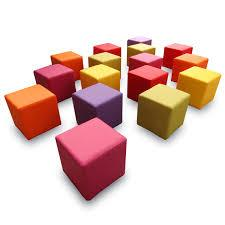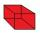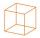# Cube

One cube has an edge increased five times. How many times will larger it's surface area and volume?

k1 =  25
k2 =  125

### Step-by-step explanation:

${k}_{1}={5}^{2}=25$
${k}_{2}={5}^{3}=125$Did you find an error or inaccuracy? Feel free to write us. Thank you!Tips to related online calculators
Check out our ratio calculator.
Tip: Our volume units converter will help you with the conversion of volume units.

## Related math problems and questions:

• Special cubeCalculate the edge of cube, if its surface and its volume is numerically equal number.
• CubeCalculate the surface cube with edge 11 dm.
• The cubeThe cube has a surface area of 486 m ^ 2. Calculate its volume.
• CubeThe sum of lengths of cube edges is 57 cm. What is its surface and volume?
• The cubeThe cube has a surface of 600 cm2. What is its volume?
• Cube 3How many times will increase the volume of a cube if we double the length of its edge?
• Rectangular cuboidThe rectangular cuboid has a surface area 5334 cm2, and its dimensions are in the ratio 2:4:5. Find the volume of this rectangular cuboid.
• CuboidHow many times will increase the volume of a cuboid, if one dimension is twice larger, second dimension three times larger and third dimension four times lower?
• CubeHow many times increases the surface area of a cube with an edge 23.4 cm if the length of the edge doubles?
• Cube 6Volume of the cube is 216 cm3, calculate its surface area.
• Cube zoomHow many percents do we increase the volume and surface of the cube if we magnify its edge by 38 %?
• Cuboid to cubeA cuboid with dimensions of 9 cm, 6 cm, and 4 cm has the same volume as a cube. Calculate the surface of this cube.
• Surface area of a cubeWhat is the surface area of a cube that has an edge of 3.5?
• Cube 2How many times will increase if the surface area of the cube if we triple length of its edge?
• The cubeThe cube has a surface area of 216 dm2. Calculate: a) the content of one wall, b) edge length, c) cube volume.
• TerezaThe cube has an area of base 256 mm2. Calculate the edge length, volume, and area of its surface.
• Cube V2SThe volume of the cube is 27 dm cubic. Calculate the surface of the cube.